# Foundations of Modern Finance II

Get the grades you deserve thru our step by step guide on your MITx MicroMasters Finance - Foundations of Modern Finance II MITx 15.415.2x

# Final Exam

Question 1
The current level of the S&P500 index is \$2004, the futures price of a contract on S&P500 maturing 3 months from now is F=\$2010. The futures contract is settled in cash with the payout at maturity equal to the closing value of the S&P500 index on that day. The 1-year continuously compunded spot risk-free rate is r_f=2.0%.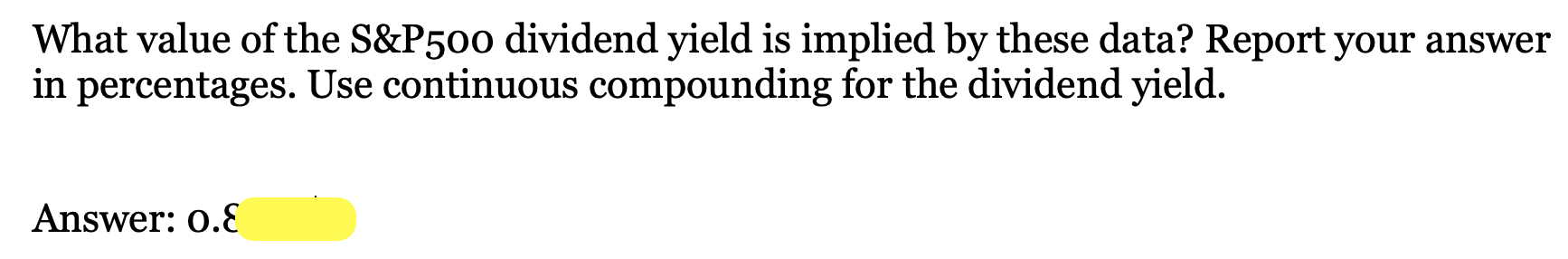# Week 20: Payout & Risk Management

KWC is a public corporation traded on NASDAQ. KWC's assets generate a perpetual, annual free cash flow of \$200 million, including this year. KWC is all equity financed and currently has 100 million shares outstanding. Since KWC has exhausted its growth opportunities, its board decides to announce its first cash dividend using its earnings from the current year (\$200 million in total). The day after the announcement, it will pay this cash dividend. (For valuation purposes, ignore this one day period.) The firm's cost of capital is 10%. Ignore corporate and personal taxes.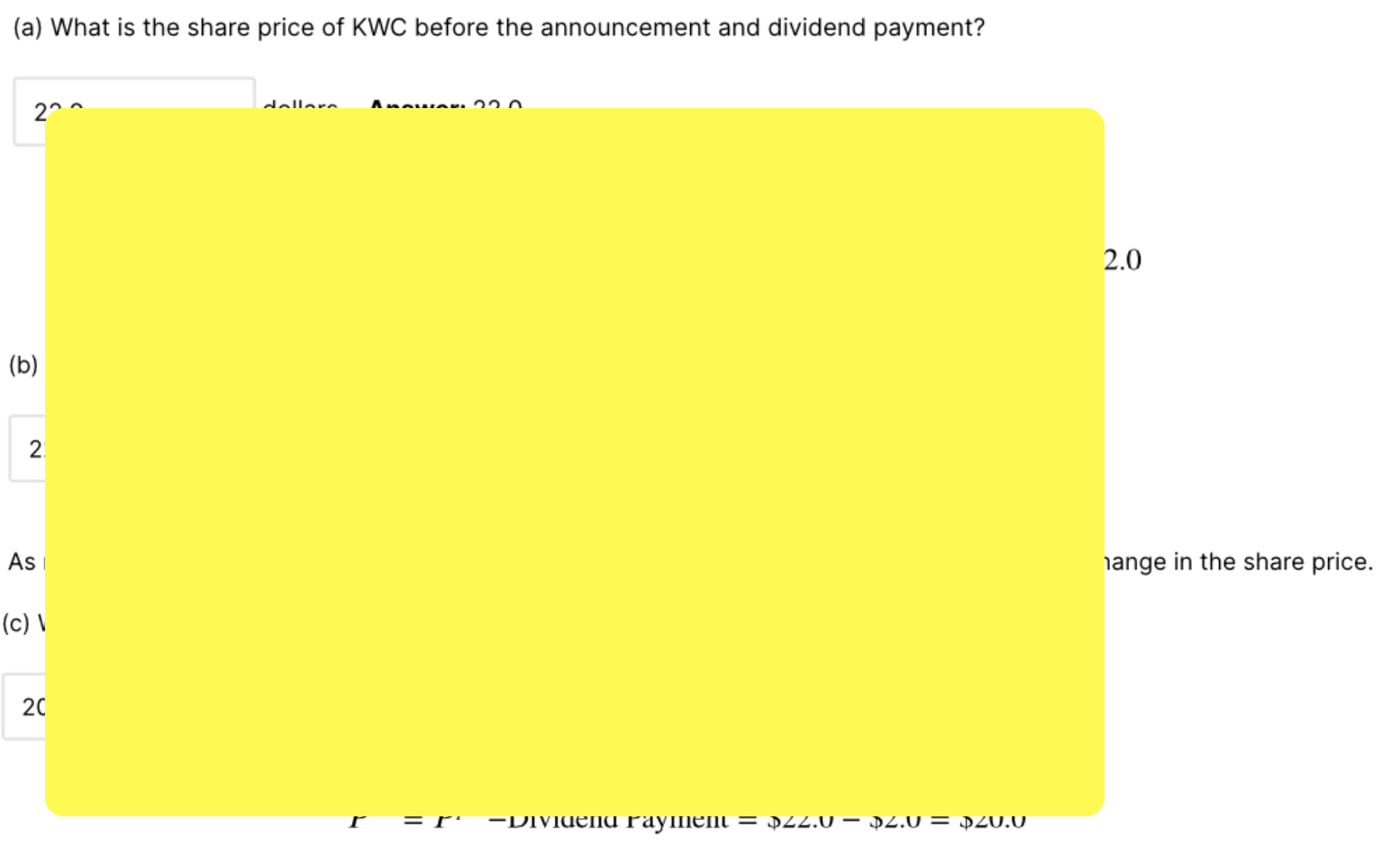# Week 19: Investment and Financing

Kurz Manufacturing is currently an all-equity firm with 16 million shares outstanding and with stock price of \$8.4 per share. Although investors currently expect Kurz to remain an all-equity firm, Kurz plans to announce that it will borrow \$55 million by issuing debt and use the funds to repurchase shares (i.e., Kurz's announcement is not anticipated by investors and thus not reflected in the current stock price). Kurz will pay interest only on this debt, and it has no further plans to increase or decrease the amount of debt. Kurz is subject to a 38% corporate tax rate.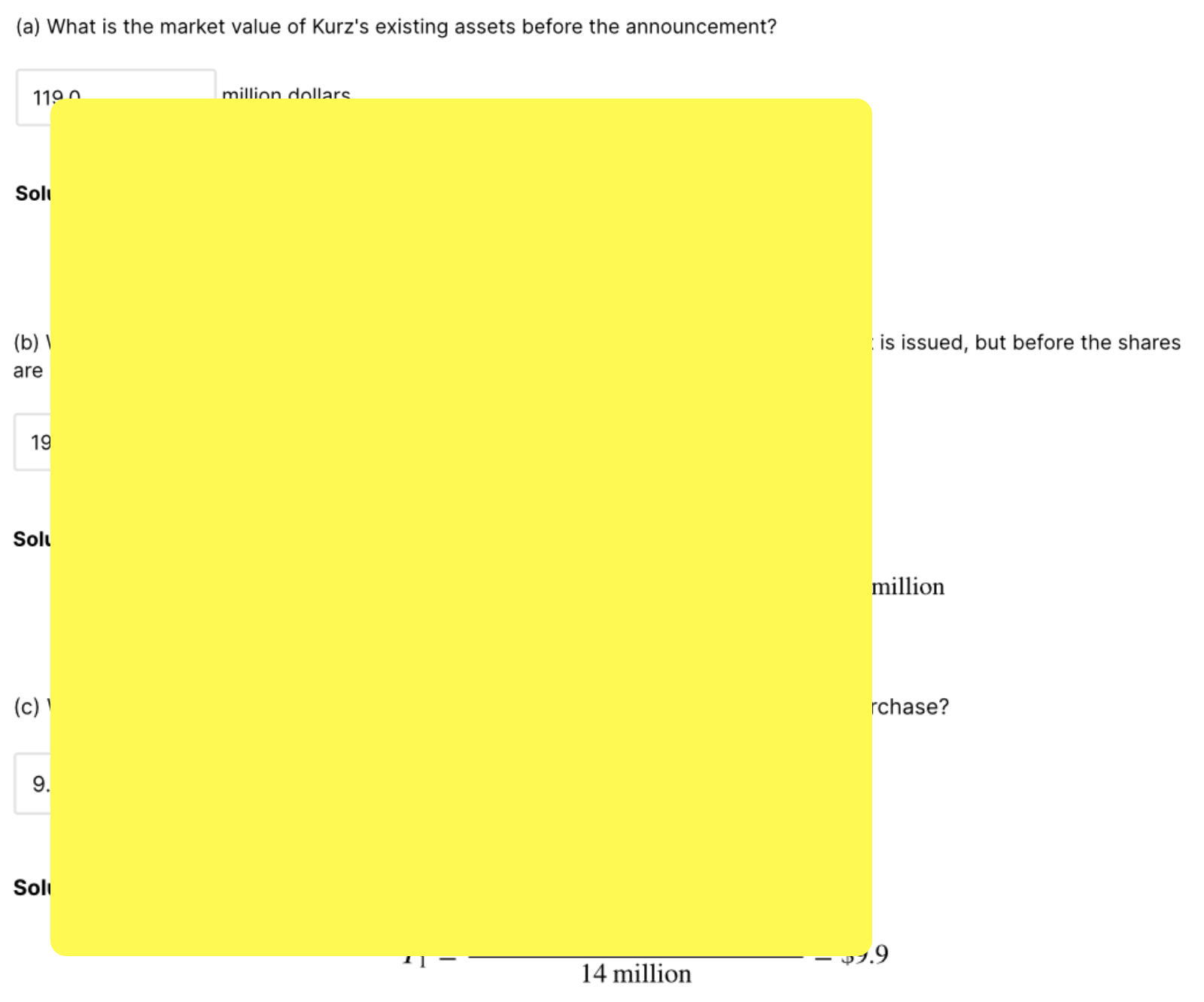# Week 18: Financing, Part II

Consider a two-period setting, year 0 and 1. All investors are risk-neutral and the risk-free rate is 0. By year 0, Bond Ryder (BR) has suffered a series of negative shocks and is faced with a grim situation. It has debt outstanding of face value \$55 million which is due next year (year 1). Next year, its assets in place have the following prospects. Assume no corporate taxes. BR’s assets yield the following payoffs in year 1: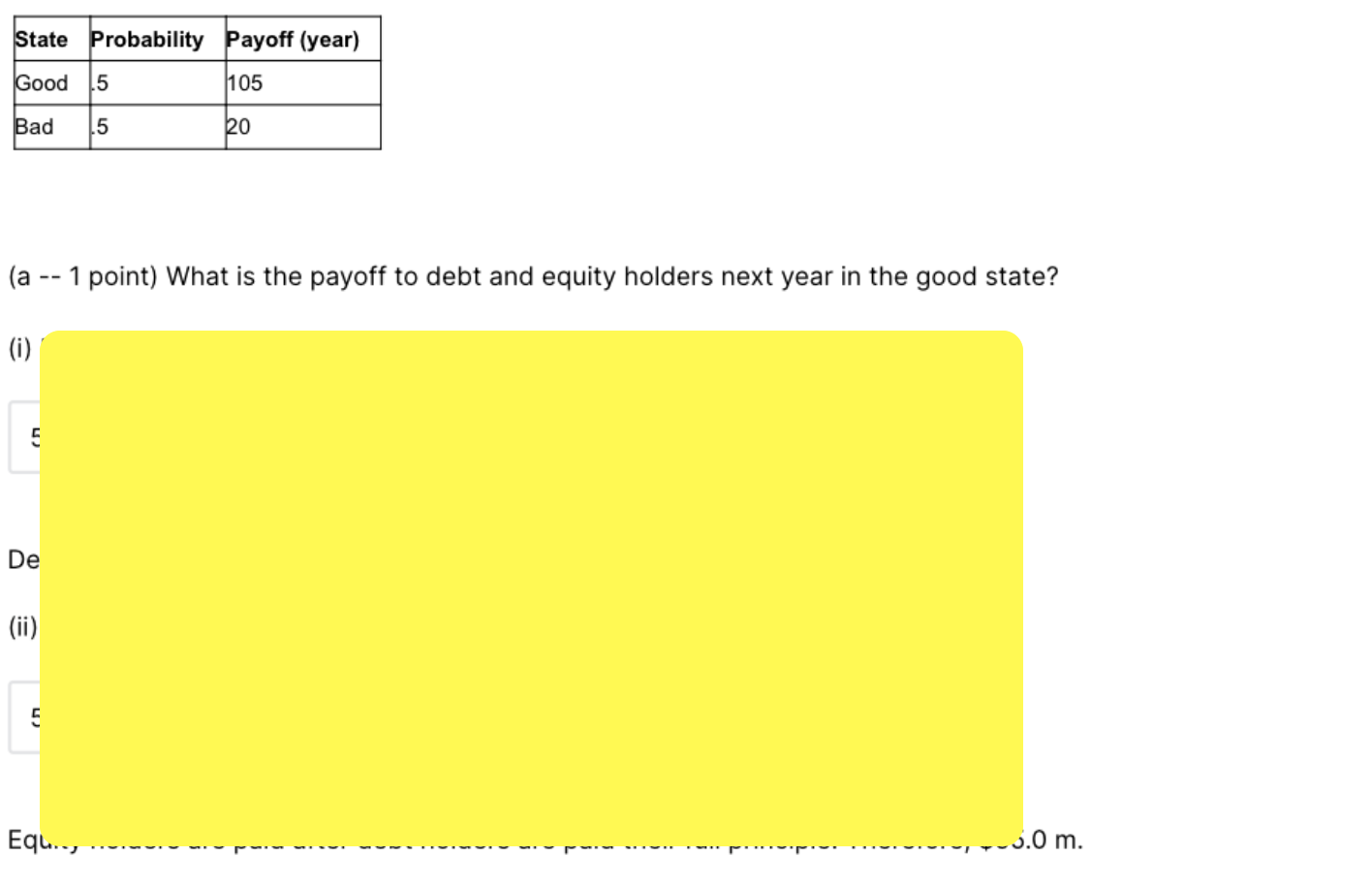# Week 17: Financing, Part I

Binomial Solutions Inc (BSI) is a financial software company. Its asset has a current market value of \$100 million (year 0). The asset value can either grow by 30% next year (year 1) with probability 63% (the up state), or decline by 30% (the down state) with probability 37%. BSI has a bond outstanding with a total due amount of \$81 million, which is due next year. Since both BSI's stock and bond are traded in the market, we also treat its asset as traded. There are 1 million shares of stocks. The current risk-free rate is 1.99%.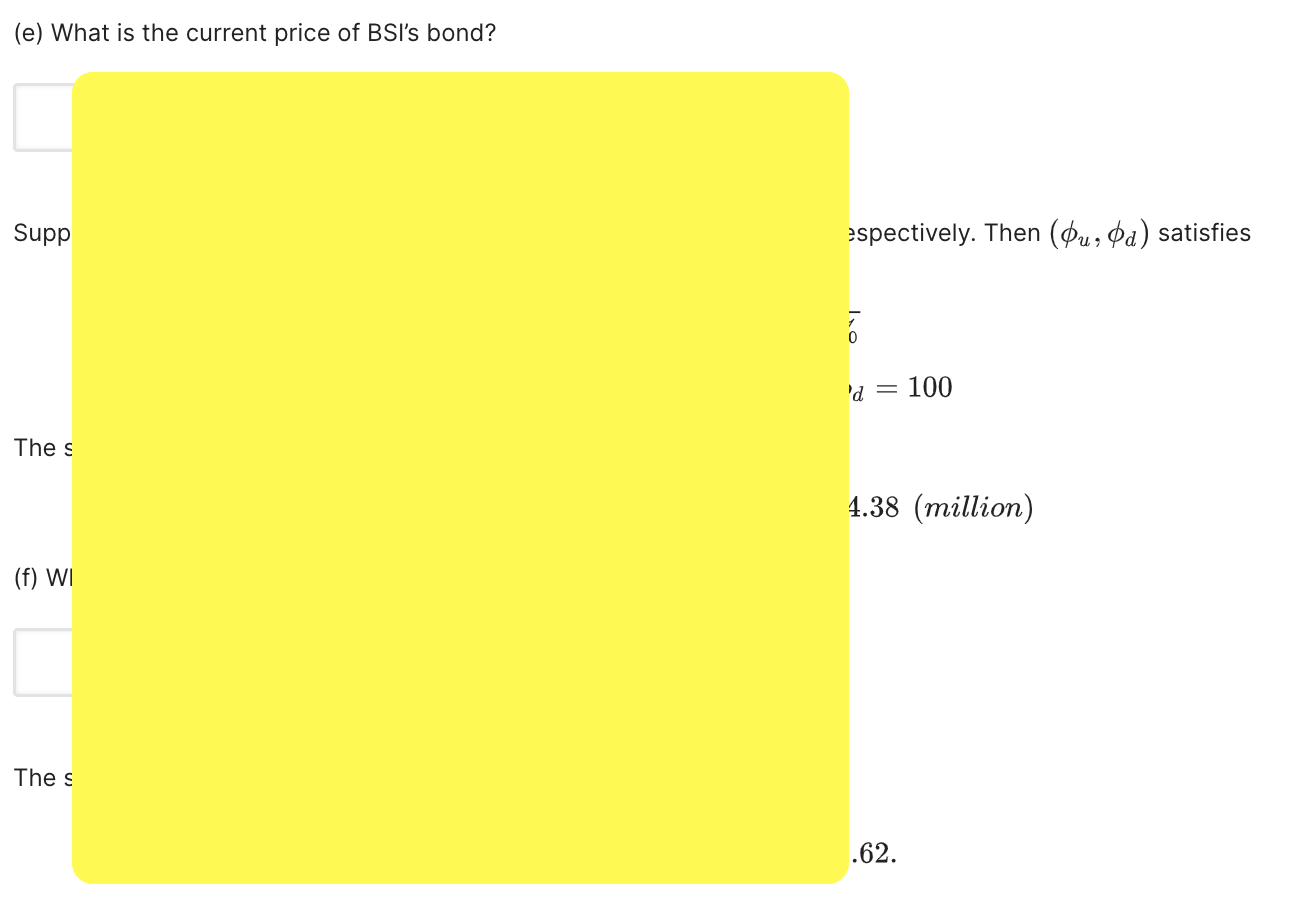# Week 16: Capital Budgeting, Part II

Bio-Egen Inc. is a technology firm working on a new technology for producing electricity. The new technology involves two stages of investment. The first stage, which starts at time 0 and takes one year to complete, requires an investment of \$30 million and has a probability of success of 16%. The second stage starts at time 1 and takes one year to complete. If the first stage is successful, the second stage has a probability of success of 76%. Otherwise, the second stage has a probability of success of only 7%. In both cases, the second stage requires an investment of \$92 million. If successful, this technology will produce a perpetual risky stream of cash flows of \$50 million per year, starting in year 2 . Assume that the project's risk is completely idiosyncratic, and thus the appropriate discount rate for all cash flows is the risk-free rate, which is 10% per year.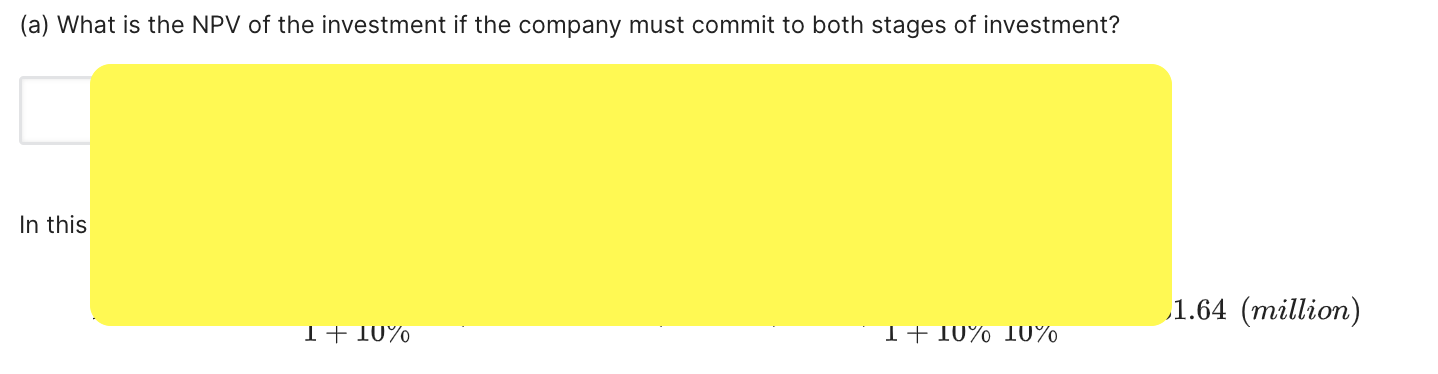# Week 15: CAPM

Problem Set 15
Suppose that all assumptions of CAPM hold. Suppose that there are only two risky assets in the market, A and B. A has expected return of r ‾_A=14%, and B has expected return of r ‾_B=10%. A has standard deviation of return of σ_A=25%, and B has standard deviation of return of σ_B=20%. Returns of A and B are uncorrelated with each other. The risk-free rate is r_f=1%.

Compute the weight of asset A in the market portfolio.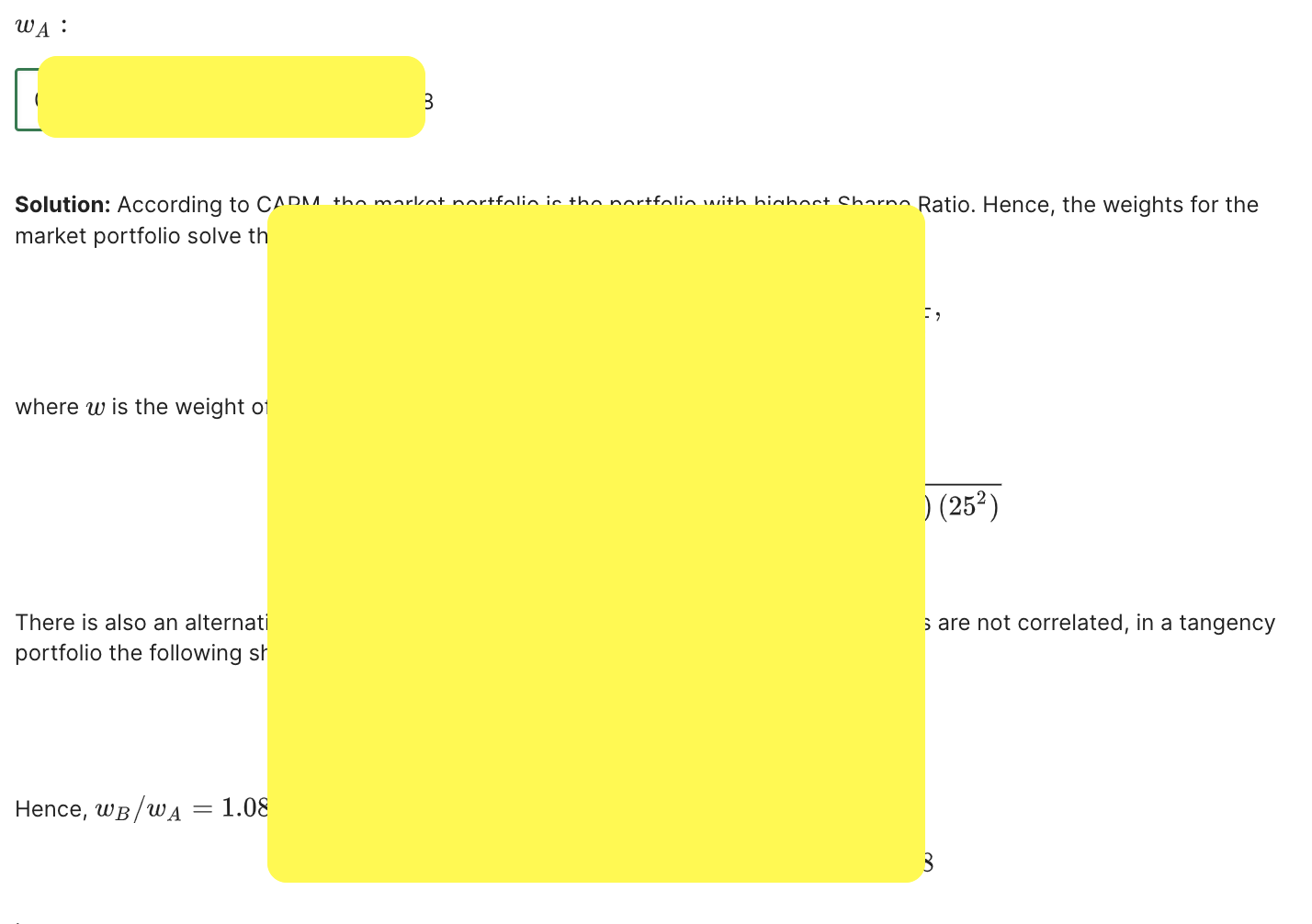# Week 14: Portfolio Theory

Problem Set 14
Suppose there are 100 risky stocks in the market, and their returns follow a single-factor model. In particular, return on stock i is given
r_i=r ‾_i+bF+ε_i

where the expected return r ‾_i=7% the factor loading b=0.188 is the same for all stocks, the factor F has zero mean and unit variance, and the standard deviation of the idiosyncratic shock ε_i is 40%. Assume that the risk-free rate r_f=1%. Consider a portfolio P with equal allocation into each of the stocks and zero weight on the risk-free asset.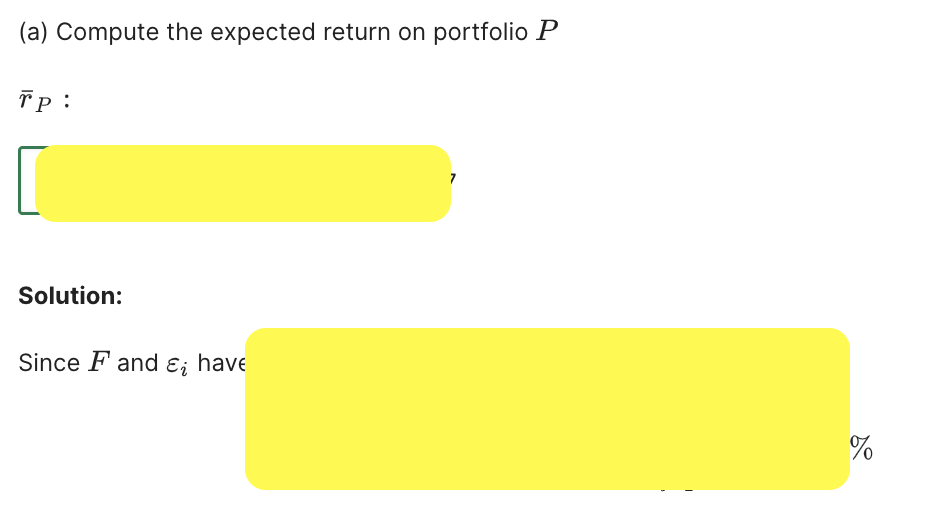# Week 13: Options, Part II

Problem Set 13
Consider a stock paying no dividends. Price movements follow the binomial model with the following tree.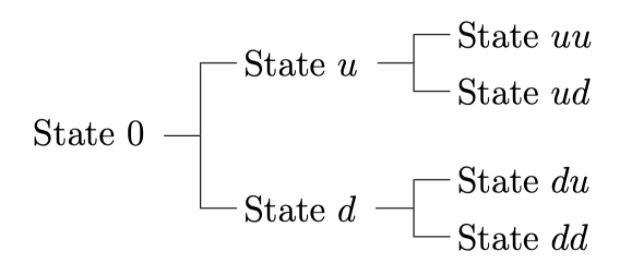with the prices at each node
Price
State 0,    S0     100
State u,    Su     196.0
State d,    Sd.     56.0
State uu, Suu   384.16
State ud, Sud   109.76
State du, Sdu.  109.76
State dd, Sdd    31.36
Interest rate is 5% per period.

What is the risk-neutral probability of the up-move at the time-0 node?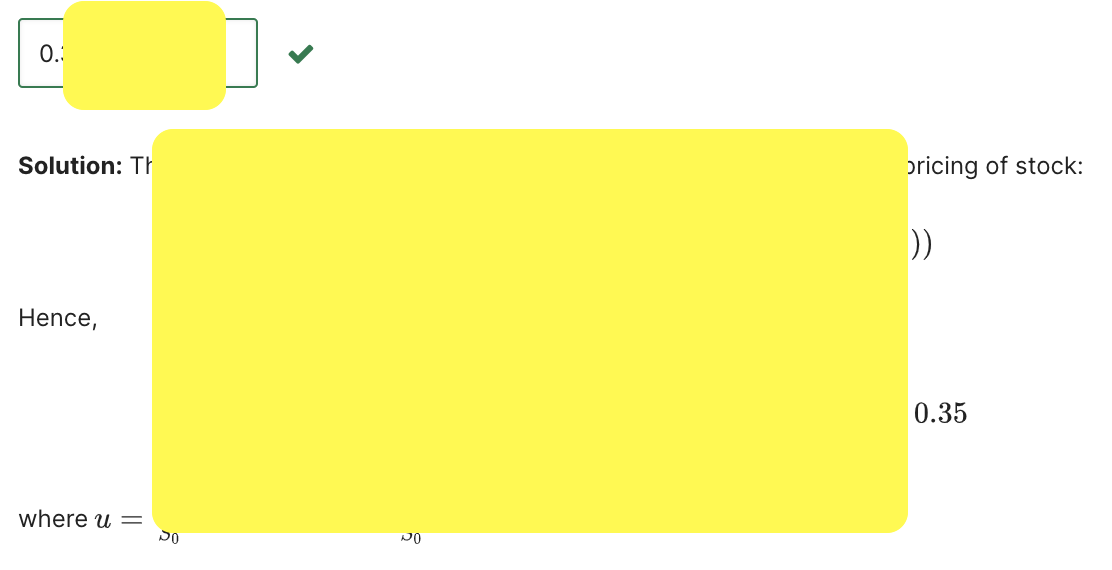# Week 12: Options, Part I

Problem Set 12
Consider two European call options on the stock of XYZ. Both options mature one year from now. The first option (Option #1) has a strike price of \$40.0 and trades at \$19.8 today; the second option (Option #2) has a strike price of \$55.0. Currently, the stock price is equal to \$55.4, and the one-year continuously-compounded risk-free rate is 3%. The stock does not pay dividends.
What is the lowest price of the second option (Option #2) consistent with absence of arbitrage?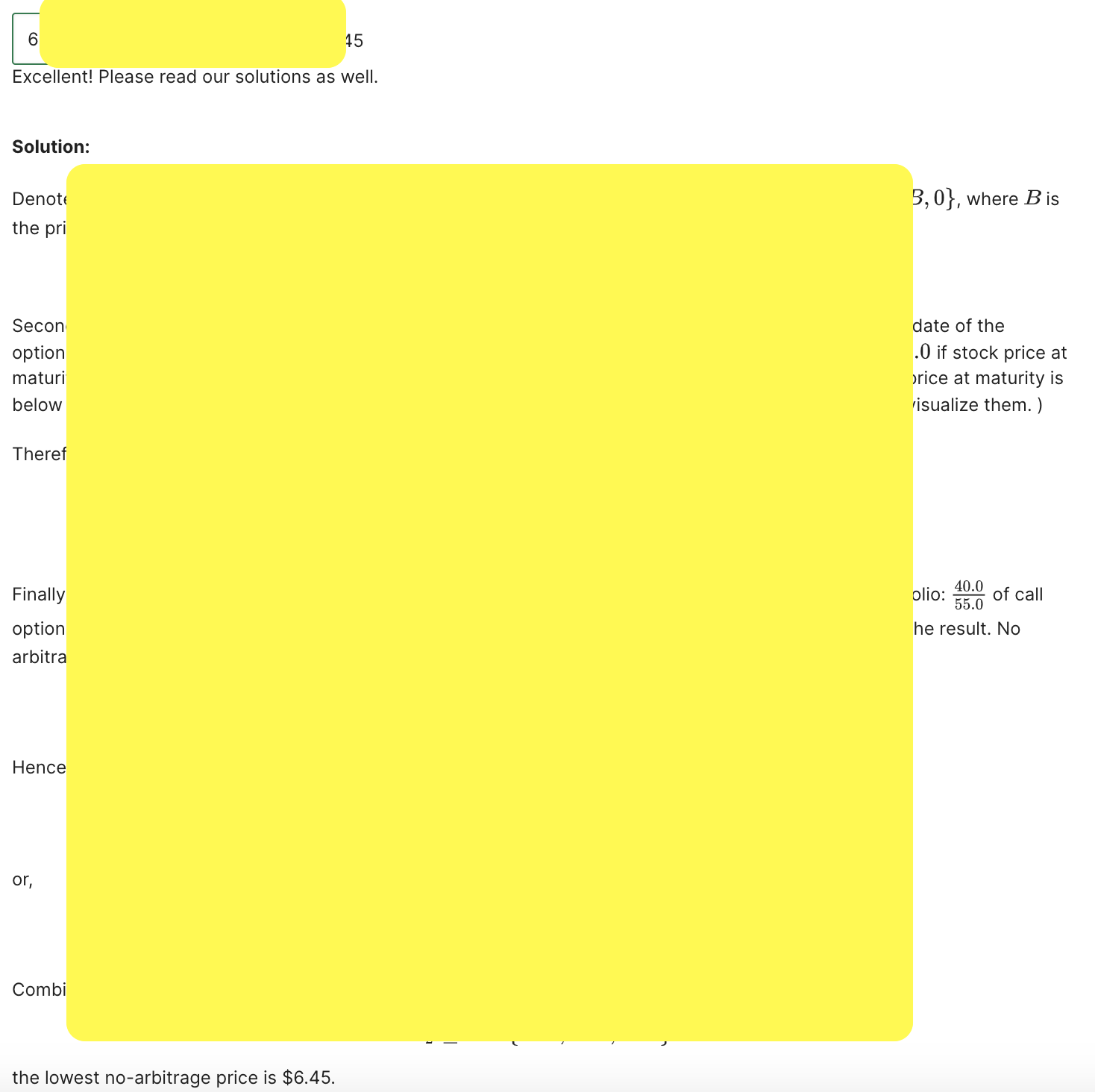# Week 11: Forwards and Futures

Problem Set 11
Consider a swap contract. The floating leg of the contract makes a payment at the end of each month, starting one month from now, equal to the spot price of natural gas at that time. The last payment on the swap contract will be 3 months from now. The fixed leg of the contract pays the same amount \$X  at the end of each month. Current futures prices for natural gas are F1 = \$39.3, F2 = 40.6, F3 = \$45.6  for the 1-, 2-, and 3-month contracts, respectively. Assume that the term structure of risk-free interest rates is flat, with the annual rate (continuously compounded) equal to rf =1%.
Compute the value of the fixed leg of the forward contract. X=?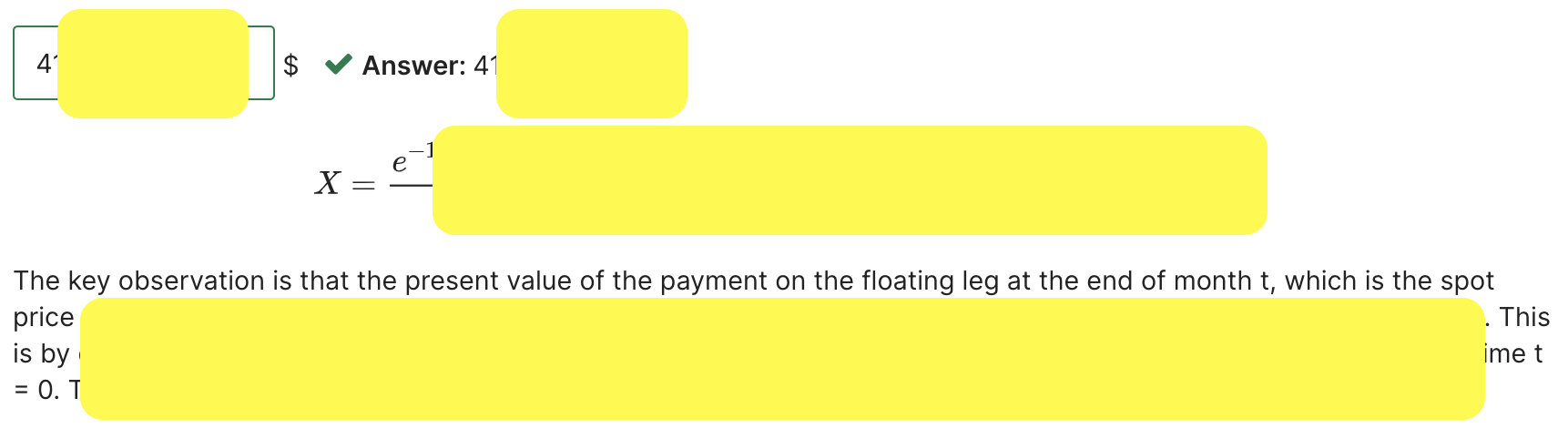# Sign up now and get more than 50% off the rack discount!

We can't tell you how we are your best tutor and the answer key to your exams and studies; however, it does have value beyond scoring in your MITx work.
In fact, if you want to get the credentials and not waste your monies for MIT's admission into the SCM program, and have the best learning experience possible, then, you need to use theexamhelper to its full potential. And that applies to the materials as well as supplemental materials – wherever theexamhelper's Solution Key that has explanations and solutions.
What Are the Benefits of Using theexamhelper's Solution Key?

There are 3 main benefits from following this process for completing and reviewing your work.
• Enhanced Understanding of the Concepts Covered
• Improved Self-teaching Skills
• Get high scores for your exams
• Become a Super Learner
• Get admitted into MIT's Masters in Applied Science in Supply Chain Management in MIT

Learners
Solutions
Hrs/Weekly
Success
Special offer

# For a limited time!

Why wait? Pay now or pay later, get the same solutions!
Exclusive
Deal
50% OFF